Back to index
Find the roots of several nonlinear equations - for the HP-42S

By Namir Shammas

RootsEqns is a program that finds the roots of N (<= 10) nonlinear functions. The program uses a straightforward version of Newton's algorithm:

x' = x - F(x) / J(x)

Where

x = array of guesses
x' = array of refined guesses
F(x) = array of nonlinear functions
J(x) = Jacobian matrix of function derivatives, J(i,j) = dFi(x)/dxj

The iterations continue until one of these conditions are met:

1. The maximum number of iterations is reached.
2. The expression ||x' - x|| / N^0.5 < XTol is true
3. The expression ||F(x)|| / N^0.5 < FTol is true
Usage:

1. To start the program execute XEQ "RTEQNS"
2. The program prompts you to enter the functions toleance value.
3. The program prompts you to enter the root-guesses toleance value.
4. The program prompts you to enter the maximum number of iterations.
5. The program displays the array of guesses in edit mode. Enter the values (you can inspect the various values and edit them). When you are done, press the R/S key.
6. The program displays the solution vector in edit mode. If the maximum number of iterations is exceeded you get a message first. Press R/S to view the current values in array of guesses.
Note: The program divides the tolerance values by the square-root of the number of equations in order to get an average that works better with the norm of the arrays of guesses and function values..

To program a new set of equations perform the following edits:

1. Set line 2 to be equal to the number of equations.
2. Edit or cretae labels 01 to 10 (as needed) to represent the nonlinear functions. Label 01 calculates the value for function F1(x). Label 02 calculates the value for function F2(x), and so on
3. In labels 01 through 10 use registers 01 through 10 to represent x(1) through x(10).
4. Edit label 36 to make sure that all of the elements of array VX are copied to memory registers 01 and up. Here is an example of label 36 copying data to solve for 2 nonlinear equations:

LBL 36
INDEX "VX"
RCLEL
STO 01
I+
RCLEL
STO 02
RTN
To copy more elements insert the following command pattern as many times needed:

I+
RCLEL
STO <
register_number>

After editing for a specific set of equations you may want to save the program under a distinct name.

Example (1):

NOTE: You can load the file RootsEqns.raw to run the next example.

Given the functions:

F1(x) = x(1)^2 + x(2)^2 - 1
F2(x) = x(1)^2 - x(2)^2 + 0.5

Find the roots near x(1) = 1 and x(2) = 1. Allow 100 iterations at most and use a root tolerance of 1E-5 and function tolerance of 1E-8.

The code for labels 01, 02, and 36 for this example are:

LBL 01
XEQ 36
RCL 01
X^2
RCL 02
X^2
+
1
-
RTN
LBL 02
XEQ 36
RCL 01
X^2
RCL 02
X^2
-
0.5
+
RTN
LBL 36
INDEX "VX"
RCLEL
STO 01
I+
RCLEL
STO 02
RTN

Also make sure that line 2 contains the number 2 which is the number of nonlinear equations.

XEQ "RTEQNS"
XTOL=          1.0000E-5
1E-5    RUN
FTOL=          1.0000E-8
1E-8    RUN
MAX=            100.0000
100.0000    RUN
X1?
1.0000    RUN
X2?
1.0000    RUN
VX=       [ 2x1 Matrix ]
1:1=              0.5000
2:1=              0.8660

The program found roots at x(1) = 0.5 and x(2) = 0.866.

Example (2):

NOTE: You can load the file RootsEqns3.raw to run the next example. Otherwise you need to perform the edits described in this example.

Given the functions:

F1(x) = x(1) + x(2) + x(3)^2 - 12
F2(x) = x(1)^2 - x(2) + x(3) - 2
F3(x) = 2 * x(1) - x(2)^2 + x(3) - 1

Make sure that line 2 contains the integer 3 (the number of nonlinear equations to solve), as shown in the next code snippet:

01 LBL "RTEQNS"
02 3
03 STO "N"

Also edit labels 01, 02, 03, and 36 to be as follows:

LBL 01
XEQ 36
RCL 01
RCL 02
+
RCL 03
X^2
+
12
-
RTN
LBL 02
XEQ 36
RCL 01
X^2
RCL 02
-
RCL 03
+
2
-
RTN
LBL 03
XEQ 36
RCL 01
2

RCL 02
X^2
-
RCL 03
+
1
-
RTN
LBL 36
INDEX "VX"
RCLEL
STO 01
I+
RCLEL
STO 02
I+
RCLEL
STO 03
RTN

After performing the above edits, you are ready to use the program.

Find the roots near x(1) = 0, x(2) = 0, and x(3) = 0. Allow 100 iterations at most and use a root tolerance of 1E-5 and function tolerance of 1E-8.

Make sure that line 2 contains the number 2 which is the number of nonlinear equations.

XEQ "RTEQNS"
XTOL=          1.0000E-5
1E-5    RUN
FTOL=          1.0000E-8
1E-8    RUN
MAX=            100.0000
100.0000    RUN
X1?
0.0000    RUN
X2?
0.0000    RUN
X3?
0.0000    RUN
VX=       [ 3x1 Matrix ]
1:1=             -0.2337
2:1=              1.3532
3:1=              3.2986

The program found roots at x(1) = -0.2337, x(2) = 1.3532, and x(3) = 3.2986.

Run the program again and provide an initial guesas of 1 for the three roots. Use the same values for the tolerances and maximum iteration limits.

XEQ "RTEQNS"
XTOL=          1.0000E-5
RUN
FTOL=          1.0000E-8
RUN
MAX=            100.0000
RUN
X1?
1.0000    RUN
X2?
1.0000    RUN
X3?
1.0000    RUN
VX=       [ 3x1 Matrix ]
1:1=              1.0000
2:1=              2.0000
3:1=              3.0000

The program found roots at x(1) = 1, x(2) = 2, and x(3) = 3.

Technical Notes:

Pseudo-code

N = number of equations ' hard coded
Redimention matrix A and arrays X, DX, and F
Input XTol, FTol, MaxIter, and array X
Iter = 0
Do
Increment Iter
If Iter > MaxIter then exit
For I = 1 to N
F(I) = FX(X, I)
FF = F(I)
For J = 1 to N
A(I,J) = FF
Next J
Next I

For I = 1 to N
XX = X(I)
H = 0.01 * (1 + ABS(XX))
X(I) = XX + H
For J = 1 to N
FF = FX(X, J)
A(J,I) = (FF - A(J,I)) / H ' Jacobian matrix elements
Next J
X(I) = XX
Next I

DX = INVERSE(A) * F ' This is a matrix operation
X = X - DX ' This is a matrix operation

Loop Until ||DX|| / N^0.5 < XTol Or ||F|| / N^0.5 < FTol

Display elements of array X

Memory usage:

MA   = Matrix of function slopes
VB   = Array of function values
VX   = Array of root guesses
VDX  = Array of root guesses refinements
FTOL = Functions tolerance
XTOL = Tolerance for root refinements
N    = Number of functions
I    = Loop control variable
J    = Loop control variable
FF   = Used
XX   = Used
H    = Increment in a guess value
MAX  = Maximum number ot iterations
ITR  = Number of iterations

R00 to R10 are used to store X(1) through X(10)
R11 = Number of digits displayed
R12 = Square root of the number of equations

Flags:

00  Display control
01  Display control
02  Control of indexing array MA

Label usage:

01  Label for function F1(x)
02  Label for function F2(x)
...
10  Label for function F10(x)

00  Main loop
11  loop
12  loop
13  loop
14  loop
15  used
16  used
17  Query display mode and digits
18  Restore display mode
20  Set I = 1 + N / 1000
21  Set J = 1 + N / 1000
30  Store value in X(I)
31  Recall X(I)
32  Store value in A(I,J) if flag 2 is clear or in A(J,I) if flag 2 is set
33  Recall A(I,J) if flag 2 is clear or recall A(J,I) if flag 2 is set
34  Store value in F(I)
35  Recall F(I)
36  Copy values of array X into memory registers 01 and up.

Binary files for emulators:
 Raw binary: rootseqns.raw, rootseqns3.raw Binary for HP-42X: rootseqns.42x, rootseqns3.42x (HP-48) rootseqns49.42x, rootseqns349.42x (HP-49)

[rootseqns:]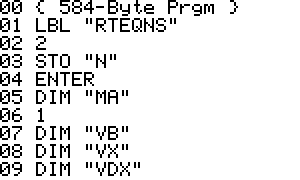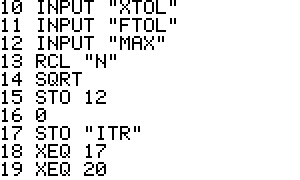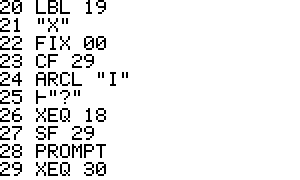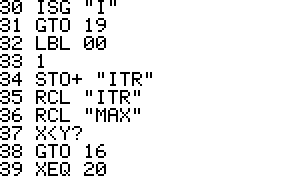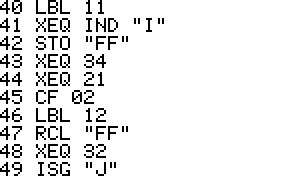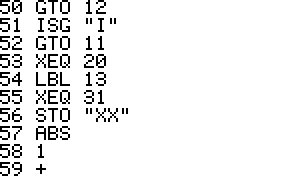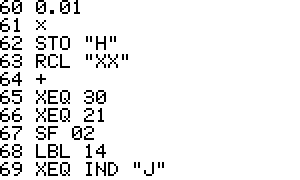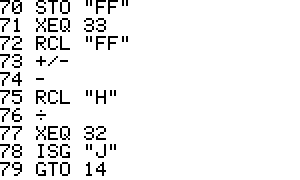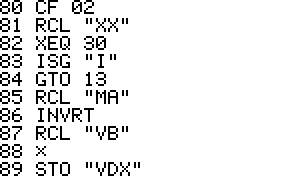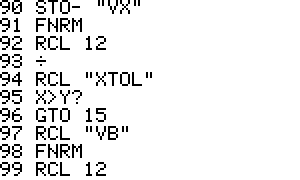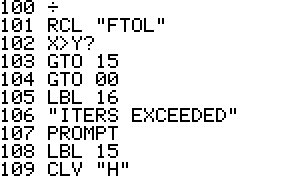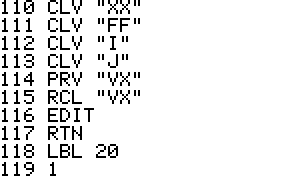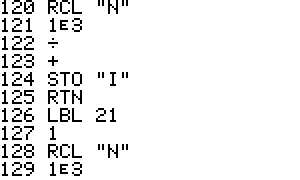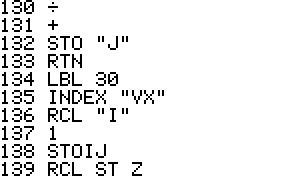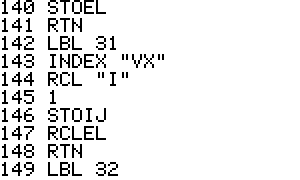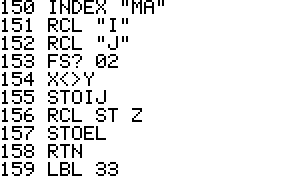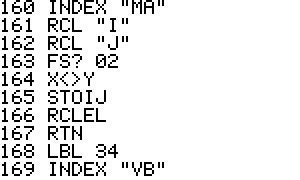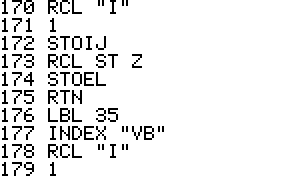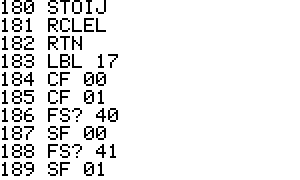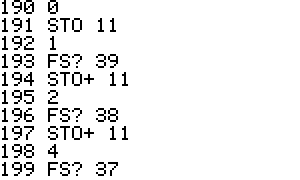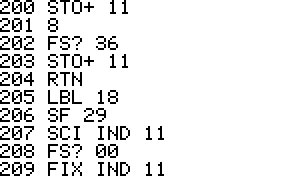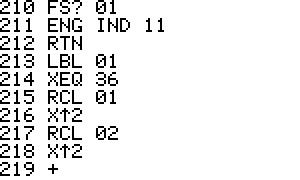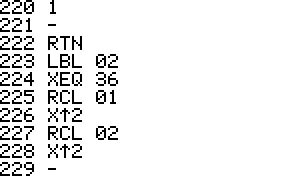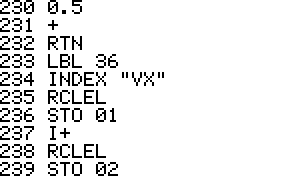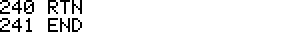[rootseqns3:]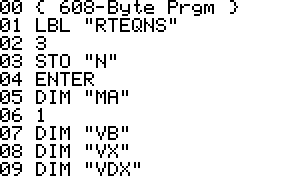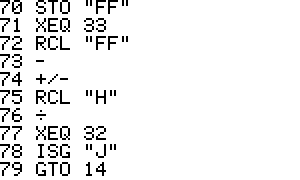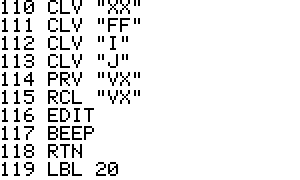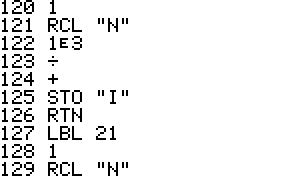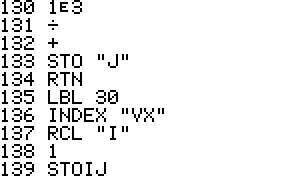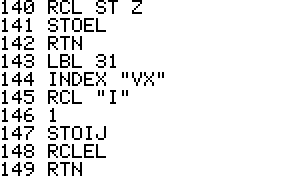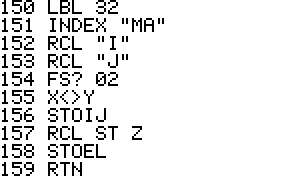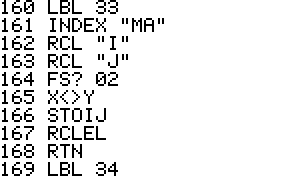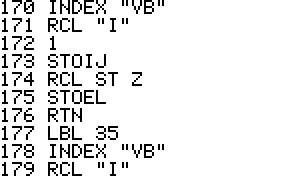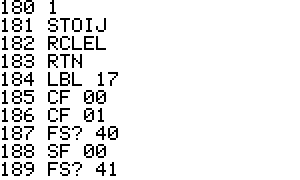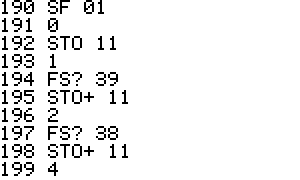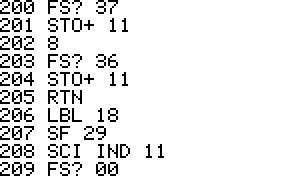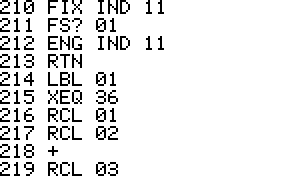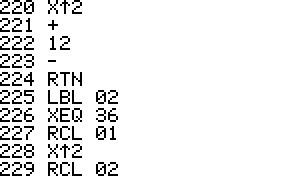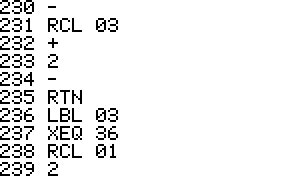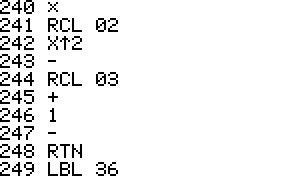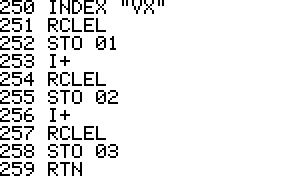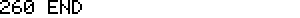Generated by 42s2html - 11 June 2005, 20:11